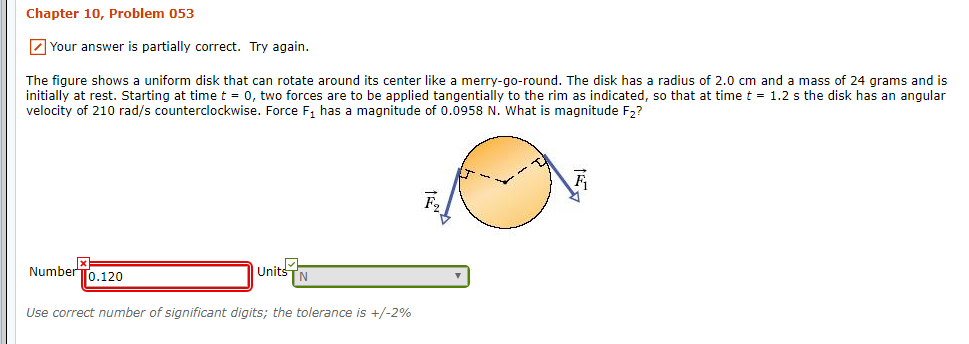# Chapter 10, Problem 053Your answer is partially correct. Try again.The figure shows a uniform disk that can rotate around its center like a merry-go-round. The disk hasinitially at rest. Starting at time t 0, two forces are to be applied tangentially to the rim as indicated, so that at time t = 1.2 s the disk has an angularvelocity of 210 rad/s counterclockwise. Force F1 has a magnitude of 0.0958 N. What is magnitude F2?radius of 2.0 cm and a mass of 24 grams and isNumberTo.120UnitsTNUse correct number of significant digits; the tolerance is +/-2%

Question
16 viewshelp_outlineImage TranscriptioncloseChapter 10, Problem 053 Your answer is partially correct. Try again. The figure shows a uniform disk that can rotate around its center like a merry-go-round. The disk has initially at rest. Starting at time t 0, two forces are to be applied tangentially to the rim as indicated, so that at time t = 1.2 s the disk has an angular velocity of 210 rad/s counterclockwise. Force F1 has a magnitude of 0.0958 N. What is magnitude F2? radius of 2.0 cm and a mass of 24 grams and is NumberTo.120 Units TN Use correct number of significant digits; the tolerance is +/-2% fullscreen
check_circle

Step 1

The moment of inertia of a disc is

Step 2

Net force acting on the system is

Step 3

Rearrange (II) in terms of ...

### Want to see the full answer?

See Solution

#### Want to see this answer and more?

Solutions are written by subject experts who are available 24/7. Questions are typically answered within 1 hour.*

See Solution
*Response times may vary by subject and question.
Tagged in

### Rotational Mechanics